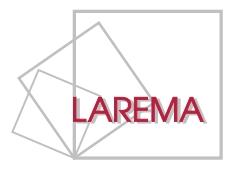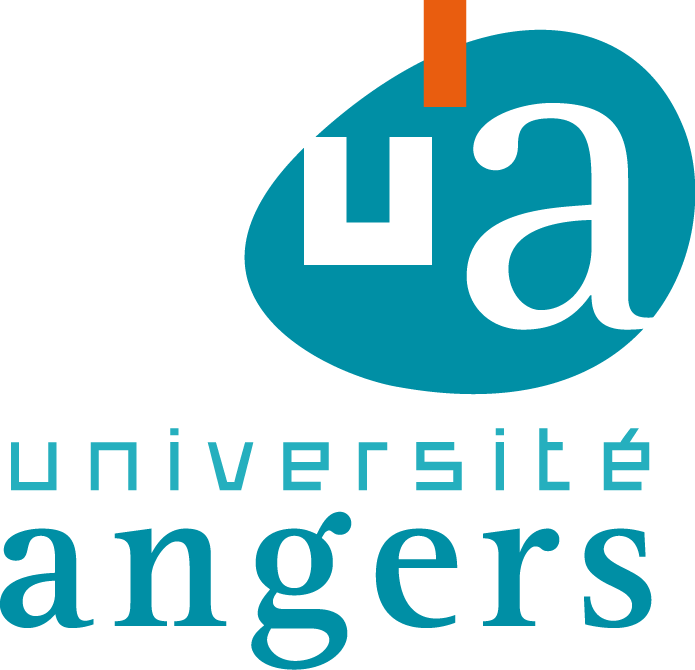# Algebraic Topology meeting## Cohomological properties of homogeneous spaces of p-compact groups

If G is a compact Lie group, and T is its maximal torus, a classical theorem by Bott and Samelson shows that the integral cohomology of G/T is torsion free and concentrated in even degrees. If G is a p-compact group, this fact is also known by using the classification of p-compact groups. We will show how to approach a proof of the Bott-Samelson result for p-compact groups without using the classification. (Joint work with W. Pitsch.)

## K(2)-local Picard groups

Picard groups can be associated with any reasonable symmetric monoidal category. In stable homotopy theory these groups are particularly interesting if one works at a prime p and considers the categories of K(n)-local spectra where K(n) is the n-th Morava K-theory at p. The corresponding Picard groups are often simply denoted Pic_n, in particular if the prime p is understood from the context. The case n=1 is well understood for all p and, for n>2, we are far from a complete understanding. In this talk I will survey the state of our knowledge on Pic_2, in particular I will describe a recent calculation of an algebraic approximation in the case p=2.

## Universal classes, cohomological finite generation and Frobenius twists

In this talk, we will present some recent developments regarding the links between two fundamental problems for the cohomology of reductive algebraic groups. The first problem is the finite generation of cohomology algebras, and the second fundamental problem is the effect of the Frobenius twist in cohomology. The link between these two problems is a certain family of classes in the cohomology of the general linear group with coefficients in the twisted divided powers of the adjoint representation.

Organizers:
Geoffrey Powell (Angers) and Lionel Schwartz (Paris 13)